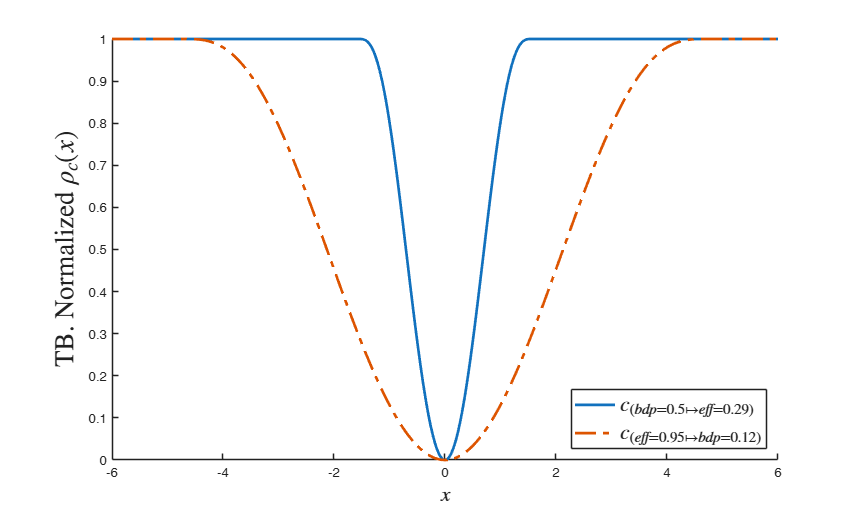# TBrho

TBrho computes rho function for Tukey's biweight

## Syntax

• rhoTB=TBrho(u,c)example

## Description

 rhoTB =TBrho(u, c) Plot of rho function.

## Examples

expand all

### Plot of rho function.

close all
x=-6:0.01:6;
rhoTB=TBrho(x,2);
plot(x,rhoTB,'LineWidth',2)
xlabel('$u$','Interpreter','Latex')
ylabel('$\rho (u,2)$','Interpreter','Latex')
text(x(1)-0.8,rhoTB(1),'c^2/6')
text(x(end)+0.2,rhoTB(end),'c^2/6')
title('$\rho (u,c)$','Interpreter','Latex')
hold('on')
c=2;
stem(c,c^2/6,'LineStyle',':','LineWidth',1)
stem(-c,c^2/6,'LineStyle',':','LineWidth',1)

## Related Examples

expand all

###Compare two rho functions for 2 different values of c.

In the first we fix the bdp (value of efficiency is automatically given), while in the second we find the efficiency (the value of bdp is automatically given)

close all
x=-6:0.01:6;
lwd=2;
hold('on')
c=TBbdp(0.5,1);
rhoTB=TBrho(x,c);
rhoTB=rhoTB/max(rhoTB);
plot(x,rhoTB,'LineStyle','-','LineWidth',lwd)
c=TBeff(0.95,1);
rhoTB=TBrho(x,c);
rhoTB=rhoTB/max(rhoTB);
plot(x,rhoTB,'LineStyle','-.','LineWidth',lwd)
xlabel('$x$','Interpreter','Latex','FontSize',16)
ylabel('TB. Normalized $\rho_c(x)$','Interpreter','Latex','FontSize',20)
legend({'$c_{(bdp=0.5 \mapsto eff=0.29)}$', '$c_{(eff=0.95 \mapsto bdp=0.12)}$'},'Interpreter','Latex','Location','SouthEast','FontSize',16)## Input Arguments

### u — scaled residuals or Mahalanobis distances. Vector.

n x 1 vector containing residuals or Mahalanobis distances for the n units of the sample

Data Types: single| double

### c — tuning parameter. Scalar.

Scalar greater than 0 which controls the robustness/efficiency of the estimator (beta in regression or mu in the location case ...)

Data Types: single| double

## Output Arguments

### rhoTB —Tukey's biweight rho associated to the residuals or Mahalanobis distances for the n units of the sample. n -by- 1 vector

function TBrho transforms vector u as follows $TBrho(u)= \left\{ \begin{array}{cc} (c^2/6) \left\{ 1-[1-(u/c)^2]^3 \right\} & |u/c| \leq 1 \\ (c^2/6) & |u/c| >1 \\ \end{array} \right.$

See equation (2.37) p. 29 of Maronna et al. (2006).

Remark: equation (2.37) is written in standardized terms in such a way that $\rho(c)=1$, so it is the previous expression divided by $(c^2/6)$

Maronna, R.A., Martin D. and Yohai V.J. (2006), "Robust Statistics, Theory and Methods", Wiley, New York.

Riani, M., Cerioli, A. and Torti, F. (2014), On consistency factors and efficiency of robust S-estimators, "TEST", Vol. 23, pp. 356-387.